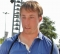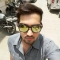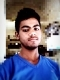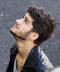## Box Stacking ProblemUpdated on 16-Jun-2020 13:33:48

638 Views

In this problem a set of different boxes are given, the length, breadth, and width may differ for different boxes. Our task is to find a stack of these boxes, whose height is as much as possible. We can rotate any box as we wish. But there is a rule to maintain.One can place a box on another box if the area of the top surface for the bottom box is larger than the lower area of the top box.Input and OutputInput: A list of boxes is given. Each box is denoted by (length, bredth, height). { (4, 6, 7), ... Read More

## Bellman–Ford Algorithm for Shortest PathsUpdated on 16-Jun-2020 13:41:56

3K+ Views

Bellman-Ford algorithm is used to find minimum distance from the source vertex to any other vertex. The main difference between this algorithm with Dijkstra’s the algorithm is, in Dijkstra’s algorithm we cannot handle the negative weight, but here we can handle it easily.Bellman-Ford algorithm finds the distance in a bottom-up manner. At first, it finds those distances which have only one edge in the path. After that increase the path length to find all possible solutions.Input and OutputInput: The cost matrix of the graph: 0  6  ∞ 7  ∞ ∞  0  5 8 -4 ∞ -2  0 ∞  ∞ ∞ ... Read More

## Check For Star GraphUpdated on 16-Jun-2020 13:50:23

408 Views

A graph is given; we have to check the given graph is a star graph or not.By traversing the graph, we have to find the number of vertices has degree one, and number of vertices, whose degree is n-1. (Here n is the number of vertices in the given graph). When the number of vertices with degree 1 is n-1 and a number of vertices with a degree (n-1) is one, then it is a star graph.Input and OutputInput: The adjacency matrix: 0 1 1 1 1 0 0 0 1 0 0 0 1 0 0 0 Output: ... Read More

## Transitive closure of a GraphUpdated on 16-Jun-2020 13:54:00

12K+ Views

Transitive Closure it the reachability matrix to reach from vertex u to vertex v of a graph. One graph is given, we have to find a vertex v which is reachable from another vertex u, for all vertex pairs (u, v).The final matrix is the Boolean type. When there is a value 1 for vertex u to vertex v, it means that there is at least one path from u to v.Input and OutputInput: 1 1 0 1 0 1 1 0 0 0 1 1 0 0 0 1 Output: The matrix of transitive closure 1 1 1 ... Read More

## Ford Fulkerson AlgorithmUpdated on 16-Jun-2020 14:01:32

3K+ Views

The Ford-Fulkerson algorithm is used to detect maximum flow from start vertex to sink vertex in a given graph. In this graph, every edge has the capacity. Two vertices are provided named Source and Sink. The source vertex has all outward edge, no inward edge, and the sink will have all inward edge no outward edge.There are some constraints:Flow on an edge doesn’t exceed the given capacity of that graph.Incoming flow and outgoing flow will also equal for every edge, except the source and the sink.Input and OutputInput: The adjacency matrix: 0 10 0 10 0  0 0  0 4 ... Read More

## Topological SortingUpdated on 16-Jun-2020 14:05:13

5K+ Views

The topological sorting for a directed acyclic graph is the linear ordering of vertices. For every edge U-V of a directed graph, the vertex u will come before vertex v in the ordering.As we know that the source vertex will come after the destination vertex, so we need to use a stack to store previous elements. After completing all nodes, we can simply display them from the stack.Input and OutputInput: 0 0 0 0 0 0 0 0 0 0 0 0 0 0 0 1 0 0 0 1 0 0 0 0 1 1 0 0 0 0 ... Read More

## Tarjan's Algorithm for Strongly Connected ComponentsUpdated on 16-Jun-2020 14:09:17

1K+ Views

Tarjan’s Algorithm is used to find strongly connected components of a directed graph. It requires only one DFS traversal to implement this algorithm.Using DFS traversal we can find DFS tree of the forest. From the DFS tree, strongly connected components are found. When the root of such sub-tree is found we can display the whole subtree. That subtree is one strongly connected component.Input and OutputInput: Adjacency matrix of the graph. 0 0 1 1 0 1 0 0 0 0 0 1 0 0 0 0 0 0 0 1 0 0 0 0 0 Output: The strongly connected ... Read MoreUpdated on 16-Jun-2020 14:12:48

1K+ Views

We know about the famous game Snake and Ladder. In this game, some rooms are present on the board, with the room number. Some rooms are connected with a ladder or with snakes. When we get a ladder, we can climb up to some rooms to reach near to the destination without moving sequentially. Similarly, when we get some snake, it sends us to a lower room to start the journey again from that room.In this problem, we have to find the minimum number of the dice throw is required to reach start to destination.Input and OutputInput: The starting and ... Read More

## Strongly Connected GraphsUpdated on 16-Jun-2020 14:16:40

2K+ Views

In a directed graph is said to be strongly connected, when there is a path between each pair of vertices in one component.To solve this algorithm, firstly, DFS algorithm is used to get the finish time of each vertex, now find the finish time of the transposed graph, then the vertices are sorted in descending order by topological sort.Input and OutputInput: Adjacency matrix of the graph. 0 0 1 1 0 1 0 0 0 0 0 1 0 0 0 0 0 0 0 1 0 0 0 0 0 Output: Following are strongly connected components in given ... Read More

## Shortest path with exactly k EdgesUpdated on 16-Jun-2020 12:34:11

344 Views

One directed graph is provided with the weight between each pair of vertices, and two vertices u and v are also provided. Our task is to find the shortest distance from vertex u to vertex v, with exactly k number of edges. To solve this problem, we will start from vertex u and go to all adjacent vertices and recur for adjacent vertices using the k value as k - 1.Input and OutputInput: The cost matrix of the graph. 0 10 3 2 ∞  0 ∞ 7 ∞  ∞ 0 6 ∞  ∞ ∞ 0 Output: Weight of the shortest ... Read More